Subsections

# Self-Consistent Field Theory

## Main Idea

Energy of Ising system is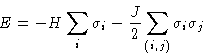It can be written as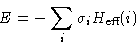with molecular field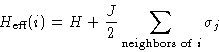We substitute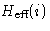by its average value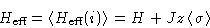and choose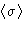from consistency condition!

## One-Particle Hamiltonian

Let us write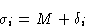The product: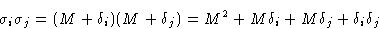Approximation: neglect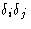. Result: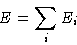with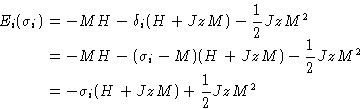(4)
We separated energy into independent contributions from each spin!

## Free energy

Partition function: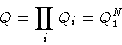Partition function for one spin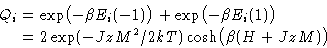Free energy: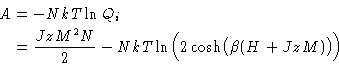(5)
This depends on the magnetization M!

## Calculation of M

There are two ways to calculate M:

### Self-Consistency Equation

By definition,. Since only Ei depends on, we have: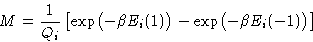or, from (4)(6)
This is a transcendent equation for M.

### Minimization of Free Energy

Free energy (5) depends on M. It should be minimal as function of M: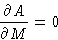orThis gives(7)
The results (6) and (7) coincide! Our theory is self-consistent.

## Critical Point and Phase Diagram

We can rewrite equation (7) as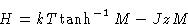(8)

1.
At large T, this is monotonic function: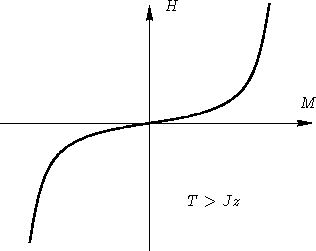This means one-phase regime

2.
At low T, this is non-monotonic function: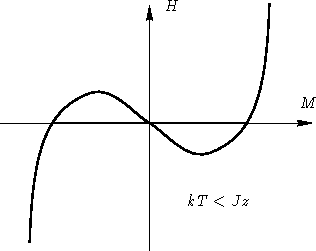From stability condition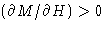we see, that this means phase separation

3.
At critical temperature Tc, we have only one point, where: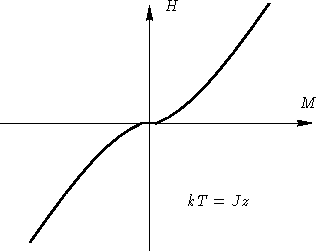At this temperature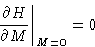or, from (8)

kT=Jz

Phase diagram: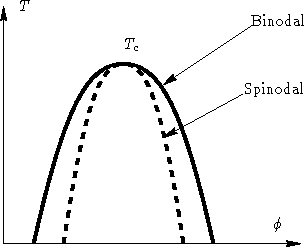## Flory Theory and Self-Consistent Field Theory

• Give same critical point
• Expansions near critical point coincide up to quadratic terms
• Give different results farther from critical point

We know how to extend it--just make a better approximation for.